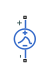# Exponential Voltage Source

Exponential pulse voltage source

• Library:
• Simscape / Electrical / Additional Components / SPICE Sources

•## Description

The Exponential Voltage Source block represents a voltage source whose output voltage value is an exponential pulse as a function of time and is independent of the current through the source. The following equations describe the output current as a function of time:

`$\begin{array}{l}{V}_{out}\left(0\le Time\le TDR\right)\right)=V1\\ {V}_{out}\left(TDR`

where:

• V1 is the Initial value, V1 parameter value.

• V2 is the Pulse value, V2 parameter value.

• TDR is the Rise delay time, TDR parameter value.

• TR is the Rise time, TR parameter value.

• TDF is the Fall delay time, TDF parameter value.

• TF is the Fall time, TF parameter value.

## Ports

### Conserving

expand all

Electrical conserving port associated with the DC current source positive voltage

Electrical conserving port associated with the DC current source negative voltage

## Parameters

expand all

Value of the output voltage at time zero, in V.

Asymptotic value of the output voltage when the output is high, in V.

Rise time delay, in s.

Time it takes the output current to rise from the Initial Value, V1 value to the Pulse Value, V2 value.

Fall time delay.

Time it takes the output current to fall from the Pulse value, V2 value to the Initial value, V1 value.

## Version History

Introduced in R2008a